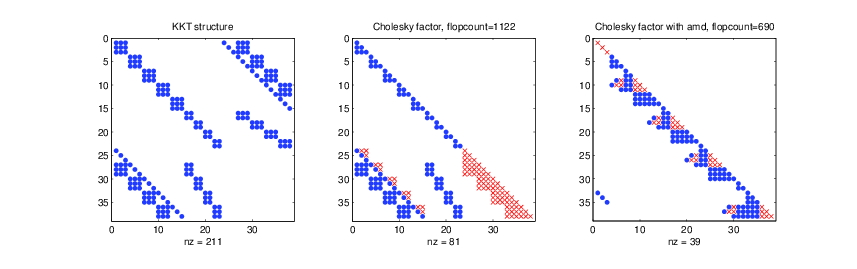{{ message }}

# ipapusha / boxqp

A primal-dual path following quadratic program solver for Matlab

## Files

Failed to load latest commit information.
Type
Name
Commit time

# boxqp

A primal-dual path following quadratic program solver for Matlab, with explicit offline factorization analysis. It is influenced by CVXOPT (coneqp) and CVXGEN. `boxqp` was written to enable Matlab simulation of MPC-style controllers and estimators, although it can solve any Quadratic Program (QP) of the form

``````minimize   (1/2)x'*P*x + q'*x
subject to A*x = b,     (dual var y)
G*x + s = h, (dual var z >= 0)
s >= 0.
``````

with variables `x`, `s` and problem data `P`, `q`, `A`, `b`, `G`, and `h`.

## Screenshot## Usage

In its simplest form, `boxqp` can be called with the following syntax:

```% set up convex problem
qp = struct;
qp.P = ...; qp.A = ...; qp.G = ...; % sparse matrices
qp.q = ...; qp.b = ...; qp.h = ...; % sparse or dense vectors

% solve problem
[fval, x, ws, status] = boxqp(qp);```

To make use of structure we do the following:

```% offline
qp  = make_mpc(A, B, z, xmin, xmax, umin, umax, Q, Qf, R, T);
ws0 = boxqp_initial(qp);
sd  = boxqp_analyze(qp, ws0);

% online
[fval, x, ws, status] = boxqp(qp, sd, ws0);```
1. `make_mpc.m` script that creates a QP description `qp`, which is a Matlab struct with fields
• `P`, `q`, `A`, `b`, `G`, `h`
2. `boxqp_initial.m` obtains an initial guess used in the path following method. The structure `ws0` contains warm-start information.
3. `boxqp_analyze.m` performs symbolic analysis of the KKT matrix and chooses the best fill-reducing permutation. The structure `sd` contains offline analysis information.
4. `boxqp.m` the solver itself

## Installation

This solver requires the command `ldlsparse(..)` which is available from the LDL package, a part of SuiteSparse.

Here is an installation procedure:

``````localhost\$ wget http://faculty.cse.tamu.edu/davis/SuiteSparse/SuiteSparse-4.5.2.tar.gz
localhost\$ tar xvzf SuiteSparse-4.5.2.tar.gz
``````

then, within Matlab:

``````> cd SuiteSparse/LDL/MATLAB/
> ldl_install
``````

This will make the command `ldlsparse(..)` available for the solver, enabling factorization caching. Use `pathtool` or `addpath` to add LDL to the Matlab path.

## Performance

### MPC problem, n = 8 (states), m = 4 (inputs), T = 20 (horizon)

Avg Solve time (ms) Rate (Hz)
naive CVX+sdpt3 2079.81 0.5
optimized CVX+sdpt3 422.88 2.4
boxqp (no structure) 21.44 46.6
boxqp (structure) 7.88 126.9
CVXGEN (Atom) 13 76.9
CVXGEN (i7) 0.97 1031

## References

• L. Vandenberghe The CVXOPT linear and quadratic cone program solvers [pdf]
• J. Mattingley and S. Boyd. CVXGEN: A Code Generator for Embedded Convex Optimization. Optimization and Engineering, 13(1):1--27, 2012 [link].

A primal-dual path following quadratic program solver for Matlab

1 tags

## Packages 0

No packages published

## Languages

You can’t perform that action at this time.